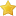• 0 Vote(s) - 0 Average
• 1
• 2
• 3
• 4
• 5
 Boolean Algebra Tutorial 1 Author Message
Hidden DragonAmazingPosts: 63
Joined: Jan 2011
Reputation: 4
 Post: #1 Boolean Algebra Tutorial 1 Welcome to my boolean algebra tutorial series. This is going to be part one of a multi-part series. I'm not sure yet how many there will be. What is boolean algebra? Well, boolean algebra is a deductive mathematical system closed over the values 0 and 1 (false and true respectively). Boolean logic is the basis for computation in binary computer systems. Any algorithm or electronic circuit can be represented by a system of boolean equations. Boolean algebra uses binary operators. A binary operator accepts a pair of boolean inputs (1 or 0, true or false) and produces a single output based on them (again 1, 0, true, false). For example, the boolean AND operator accepts two inputs, ANDs them, and produces the result. Postulates of boolean algebra: Closure: the boolean system is closed with respect to a binary operator if for every pair of boolean values it produces a single boolean result. example: Logical AND is closed because it accepts only boolean operands and produces only boolean results. Commutativity: A binary operator * is commutative if A*B = B*A for all possible values of A and B. Associativity: A binary operator * is associative if (A*B)*C = A*(B*C) for all possible values of A, B, and C. Distribution: Two binary operators * and ` are distributive if A*(B`C) = (A*B)`(A*C) for all A, B, and C values. Identity: A boolean value I is an identity element with respect to another operator * if A*I = A Inverse: A boolean value I is the inverse element with repect to an operator * if A*I=B and B does not = A (meaning B is the opposite of A). Now that we have the postulates covered, lets cover the usual operators. The symbol * represents the logical AND operation. A*B is the result of logically ANDing boolean values A and B. The symbol + represents the logical OR operation. A+B is the result of logically ORing boolean values A and B. The symbol ' is logical NOT. ` is unary meaning that it has only one operand. A' is the result of NOTing A boolean value A. The order of operations in boolean algebra are also important. The order goes parenteses (), NOT ', AND *, then OR +. I really lied about the postulates. Those weren't all of them. These postulates cover specific operators though so they are a bit different. Boolean Algebra is closed under AND, Or, and NOT The identity element with repect to * is 1 and to + is 0. There is not identity element with ' The * and + operators are commutative * and + are distributive with repect to each other Ex. A*(B+C)=(A*B)+(A*C) and A+(B*C)=(A+B)*(A+C) For every value of A there is a A' so A*A'=0 and A+A'=1. Those are all the postulates. We do, however, also have theorums that we have to cover. There are 16 important theorums. 1. A+A=A 2. A*A=A 3. A+0=A 4. A*1=A 5. A*0=0 6. A+1=1 7. (A+B)'=A'*A' 8. (A*B)'=A'+B' 9. A+A*B=A 10. A*(A+B)=A 11. A+A'*B=A+B 12. A'*(A+B')=A'*B' 13. A*B+A*B'=A 14. (A'+B')*(A'+B)=A' 15. A+A'=1 16. A*A'=0 Those are all 16 theorums that we're going to need. I know it can be a lot to take in at once so read over and review this so far. Next up we're going to be building boolean truth tables. (This post was last modified: 01-30-2011 02:29 AM by Hidden Dragon.)
01-29-2011 09:53 PM
IronsideSite OwnerPosts: 170
Joined: Nov 2010
Reputation: 7
 Post: #2 RE: Boolean Algebra Tutorial 1 The exact same thing we have this year in 2nd grade of middle school, except that we never took those 16 postulates. We only have 7 logical functions: - Not, - And, - Or, - Nand, - Nor, - Xor, - Xnor. (This post was last modified: 01-29-2011 10:17 PM by Ironside.)
01-29-2011 10:17 PM
Hidden DragonAmazingPosts: 63
Joined: Jan 2011
Reputation: 4
 Post: #3 RE: Boolean Algebra Tutorial 1 Well NAND, NOR, and XNOR are actually different gates in electrical engineering so I'll be talking about them in later tutorials. I don't include XOR in there because you can just OR an equation and then OR the inverses, then finally AND both of those answers.
01-30-2011 02:23 AM
IronsideSite OwnerPosts: 170
Joined: Nov 2010
Reputation: 7
 Post: #4 RE: Boolean Algebra Tutorial 1 Alright then.
01-30-2011 11:06 AM
JcapersNew MemberPosts: 2
Joined: Oct 2012
Reputation: 0
 Post: #5 RE: Boolean Algebra Tutorial 1 AB+A(B+C)+B(B+C)=
10-20-2012 03:09 AM
JcapersNew Member